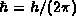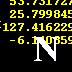Next: Index of figures Up: Atomic Reference Data for Electronic Structure Calculations Previous: References

# Summary of notation and data format

## System of units

All data in the tables is given in Hartree energy units, in which numerical values of unity are assigned to the mass m and charge e of the electron and to the reduced Planck's constant. In these units, the speed of light, c, is given by the inverse of the fine structure constant. We have used the 1986 CODATA recommended value of the fine structure constant to obtain the numerical value, c = 137.0359895, used in the RLDA AND ScRLDA calculations.

## Presentation of numerical data, element by element

The header line for each element takes the form
```Atomic number      Chemical symbol      Ground electronic configuration
```
Four separate cases are then presented in successive columns:
• Local density approximation (LDA),
• Local spin density approximation (LSD),
• Relativistic local density approximation (RLDA)
• Scalar relativistic local density approximation (ScRLDA).
Each column begins with the total energy in the given approximation and its decomposition into four parts:
``` Etot  =    Total energy
Ekin  =    Kinetic energy
Ecoul =    Electron-electron interaction energy (Hartree term)
Eenuc =    Electron-nucleus interaction energy
Exc   =    Exchange-correlation energy
```

These energies are then followed by a list of orbital eigenvalues. The orbital labels (e.g. 1s) are given to the left of all columns. The orbital labels and eigenvalues are presented in alternating boldface and plain type for ease of reading.

Conventions for orbital eigenvalues:
The LDA and ScRLDA approximations.
These have only a single orbital eigenvalue for each (nl), which is labelled on the left.
The LSD approximation.
For each (nl) there are two orbital eigenvalues, one for spin up and one for spin down. They are presented here in spin order, i.e. the first entry for each (nl) corresponds to spin down, the second to spin up. Note that "down" is the direction of the majority spin.
The RLDA approximation.
This has a single eigenvalue for each s orbital, but for non-zero l there are two eigenvalues, corresponding to the values of the sum of spin and orbital angular momenta j = l ±1/2. The first entry for a given (nl) in each column corresponds to j = l - 1/2 , the second to j = l + 1/2 .

## Presentation of numerical data in files

Those who wish to obtain all data in these tables may retrieve a compressed archive version of the original files. When decompressed and untarred, the archive, dftdata.tar.gz yields a directory containing one file and four subdirectories:
• dftdata/configurations
• dftdata/LDA/
• dftdata/LSD/
• dftdata/RLDA/
• dftdata/ScRLDA/
The configurations file contains the electronic configuration information presented elswhere in this document. The directories each contain two subdirectories:
• cations/
• neutrals/
and each of these in turn contains the individual data files (92 files for atoms, 91 for cations).
The data file name format, common to all directories, is "Atomic number""Chemical symbol", e.g. 01H, 02He, 03Li, etc. For example, the fully qualified path name of the data file for neutral uranium treated in the RLDA approximation will be:
```     dftdata/RLDA/neutrals/92U     (show contents)
```

This file name convention was facilitates simple report generation by standard Unix commands; some examples are shown below.
The essential information within each data file is identical to that described above. The first five lines contain the values ofEtot, Ekin, Ecoul, Eenuc, and Exc, with obvious labels; each subsequent line contains an orbital eigenvalue with a label. The order of the orbital eigenvalues is identical to that described above, but the labelling scheme is different:
The LDA and ScRLDA approximations.
These have only one orbital eigenvalue for each (nl), and so the conventional spectroscopic notation is used as the label: 1s, 2s, 2p,, etc.
The LSD approximation.
For each (nl) there are two orbital eigenvalues, one for spin down and one for spin up. These are labelled as nlD and nlu, i.e. 1sD, 1su, 2sD, 2su, etc. The capitalization of D is a mnemonic device to emphasize that "down" spins constitute the majority.
The RLDA approximation.
For each (nl), we use the label nlP to designate the orbital with j = l + 1/2, and nlM for the orbital with j = l - 1/2 (mnemonic for Plus and Minus). The P notation is retained for s orbitals even though it is redundant.

## Simple report generation

Our data file name convention facilitates report generation by standard Unix commands. Some simple examples follow:
Generate list of LDA 3d orbital eigenvalues, ordered by Z
With LDA/ as the current directory, execute
grep "3d" * | awk '{print \$2}'
Generate table of Hartree energies for all approximations, ordered by Z
With LDA/ as the current directory, execute
foreach file (*)
echo \$file | awk '{printf("%-6s", \$1)}'
grep "Ecoul" \$file | awk '{printf("%14.6f", \$3)}'
grep "Ecoul" ../../LSD/neutrals/\$file | awk '{printf("%14.6f", \$3)}'
grep "Ecoul" ../../RLDA/neutrals/\$file | awk '{printf("%14.6f", \$3)}'
grep "Ecoul" ../../ScRLDA/neutrals/\$file | awk '{printf("%14.6f\n", \$3)}'
endReturn to periodic tableNext: Index of figures Up: Atomic Reference Data for Electronic Structure Calculations Previous: References